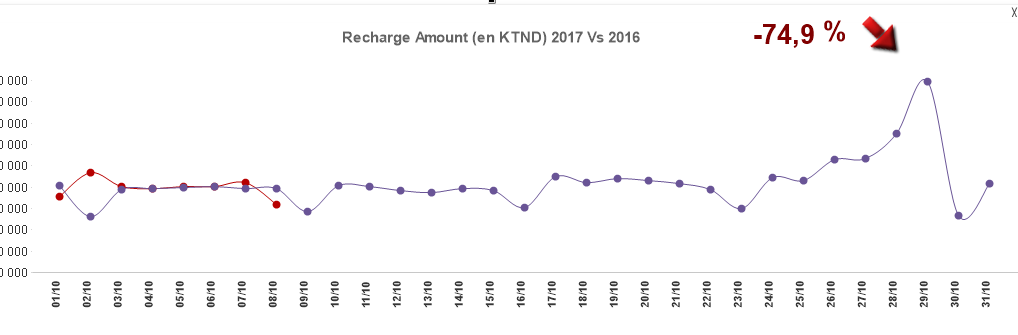# QlikView App Development

Discussion Board for collaboration related to QlikView App Development.

Announcements
Modernize Your QlikView Deployment webinar, Nov. 3rd. REGISTER
cancel
Showing results for
Did you mean:
HighlightedCreator III

## variation until today

Hello Guys,

In the graph bellow, I am comaring the recharge amount 2016 Vs 2017 and I am calculating the variation 2017 par rapport 2016

value 2017 - value 2016  / value 2016 *100 , the problem is whene the selected month is  october , the variation is false because the rest of value of october 2017 are not yet available. so i need to display the variation to be until today whene the month is october, any idea ?the variation expression :

=num((num(sum( {\$<[Year of date]={\$(=Max([Year of date]))}>} recharge_amount),'# ##0')- num(sum( {\$<[Year of date]={\$(=Max([Year of date])-1)}>} recharge_amount),'# ##0')) / num(sum( {\$<[Year of date]={\$(=Max([Year of date])-1)}>} recharge_amount),'# ##0') *100,'# ## ')

Thank you so much

3 Replies
HighlightedMVP

This?

If(GetFieldSelections(MonthField) = 'October',

num((num(sum( {\$<[Year of date]={'\$(=Today())'}>} recharge_amount),'# ##0')- num(sum( {\$<[Year of date]={'\$(=AddYears(Today(),-1))'}>} recharge_amount),'# ##0')) / num(sum( {\$<[Year of date]={'\$(=AddYears(Today(),-1))'}>} recharge_amount),'# ##0') *100,'# ## '),

num((num(sum( {\$<[Year of date]={\$(=Max([Year of date]))}>} recharge_amount),'# ##0')- num(sum( {\$<[Year of date]={\$(=Max([Year of date])-1)}>} recharge_amount),'# ##0')) / num(sum( {\$<[Year of date]={\$(=Max([Year of date])-1)}>} recharge_amount),'# ##0') *100,'# ## '))

Before develop something, think If placed (The Right information | To the right people | At the Right time | In the Right place | With the Right context)
HighlightedMVP

You can use set analysis to restrict your chart to show up until today...what is your date field called here? and what is it's format?

HighlightedCreator III

my date dimension is : Date(SetDateYear([Calendar date], Year(Today())), 'DD/MM')